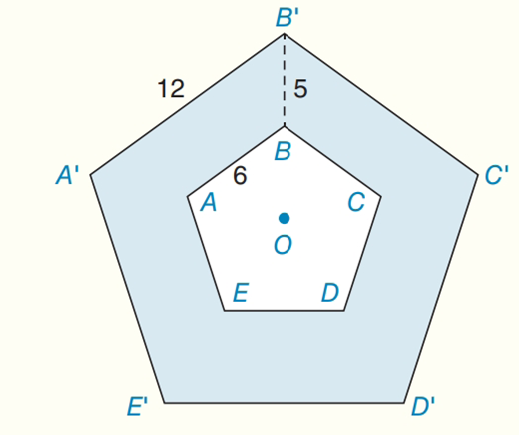Chapter 8.CR, Problem 41CRElementary Geometry For College St...

7th Edition
Alexander + 2 others
ISBN: 9781337614085

Solutions

Chapter
SectionElementary Geometry For College St...

7th Edition
Alexander + 2 others
ISBN: 9781337614085
Textbook Problem

Regular pentagons ABCDE and A ' B ' C ' D ' E ' are centered about point O, as shown. With A ' B ' = 12 , A B = 6 , and B B ' = 5 , find the area of the shaded region.To determine

To find:

The area of the shaded region.

Explanation

Formula:

The area of regular pentagon A=145(5+25)a2

Where a is the length of side of the pentagon.

Calculation:

Consider the following diagram:

To find the area of the regular pentagon ABCD:

A=145(5+25)a2

a = 6

A=145(5+25)(6)214(6.88)(36)(6.88)(9)61

Still sussing out bartleby?

Check out a sample textbook solution.

See a sample solution

The Solution to Your Study Problems

Bartleby provides explanations to thousands of textbook problems written by our experts, many with advanced degrees!

Get Started

Find f'(x) and f"(x). f(x)=x21+ex

Calculus: Early Transcendentals

What is the value of (X + 1) for the following scores: 0, 1, 4, 2? a. 8 b. 9 c. 11 d. 16

Essentials of Statistics for The Behavioral Sciences (MindTap Course List)

True or False: If a function is not increasing on an interval, then it is decreasing on the interval.

Study Guide for Stewart's Single Variable Calculus: Early Transcendentals, 8th